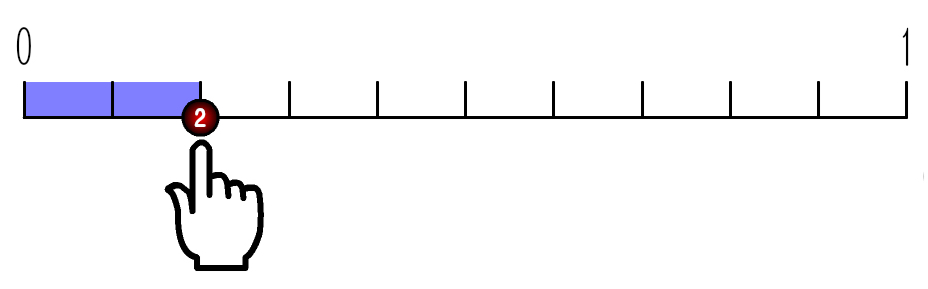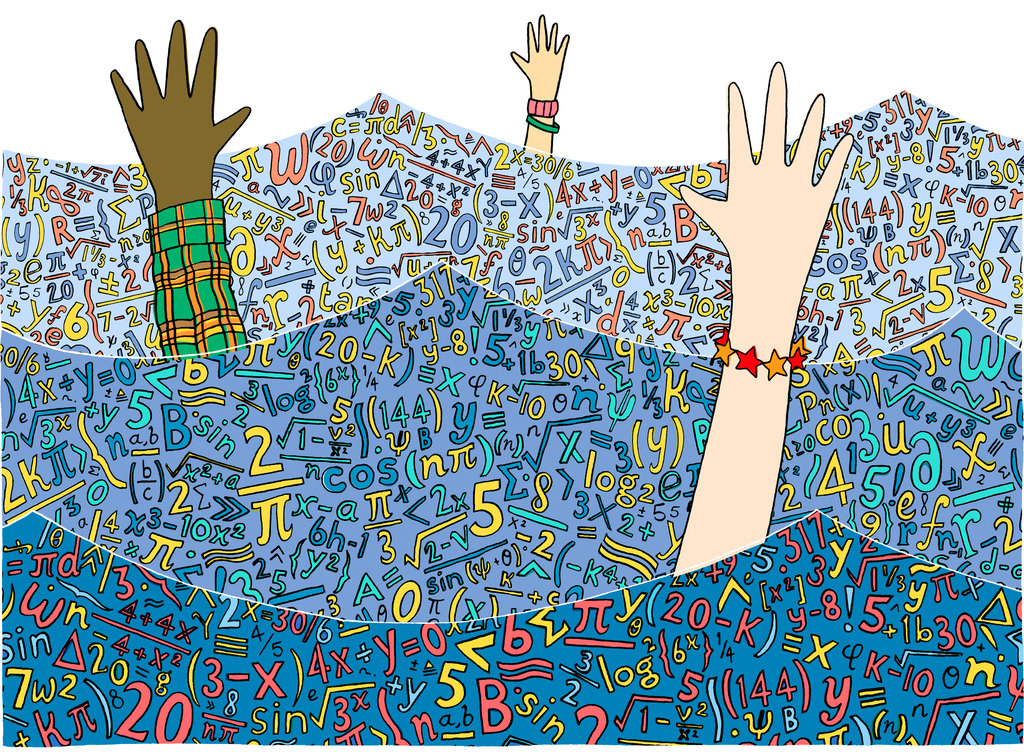# Why is mathematics so hard for some children?

In this article we’re going to discuss the difficulty of Math, but before you read ahead, I’d like to give you some notes.

• Mathematics can be roughly divided into three categories: geometry, arithmetic/algebra, and formal mathematics. This article focuses on arithmetic/algebra.
• This post talks about the difficulty of children who fail to solve even simple Math problems like “3x+3=2x find x”. Still the findings we will see in this post are definitely related with solving more difficult Math problems.
• We don’t discuss emotional factors here. But confusion is one of the biggest factors to encourage children to hate Math, so it is worthwhile to think what makes them confused.

# What is the factor of difficulty?

Although we can think of various factors, such as study habit, family, teacher, and so on, as the reason some children become terrible at mathematics, here we will focus on the factor rooted in the characteristics of mathematics itself.

To be straight to the point, the factor of the difficulty of learning mathematics is that children repeatedly experience a situation where they face mathematical concepts or notations against their existing knowledge or belief which has been successful in previous stages.Source: http://keywordsuggest.org/184982-obstacles.html

It can be truly seen around every corner of Math.
I’ll explain it more specifically, taking an example of “multiplication of decimals”, which is one of the most difficult topics for children.

# Multiplication of Decimals

Decimals are typically introduced as fractional magnitudes when measuring objects.
For example, 0.2, is learned as “two pieces of size equal to 1 divided by 10″So those who understand it and decimal numeration system and have been used to arithmetic operations on natural number could correctly operate those problems like: 0.3+0.4; 0.3×3.
It could make sense for them by applying their existing knowledge about operations on whole numbers to the problems.

However when they face operations like 0.3×0.4, It could make them upset.
Those who understand multiplication is like “a×b means b sets of the amount of a”, encounter uncertain meaning of “0.4 sets…?”.

Then children who couldn’t appropriately grasp the meaning of multiplication of decimals would drive themselves to learn by rote the rule: “calculate by writing as same way as whole numbers, then shift decimal point” without understanding.

# Variable Expression

Next, we will look at another example.
Many children tend to have difficulties when they start to learn algebra from arithmetic.
That’s because their existing belief enhanced through learning arithmetic could turn to be obstacles to learn algebra.Source: http://www.nytimes.com/2012/07/29/opinion/sunday/is-algebra-necessary.html

For instance, calculations in arithmetic always have their “answer” which is invariably a number with no operator symbol.
2+2 is “4”, 2.487×23 is “57.201”, and the area of a circle with the radius of 4.76 is “71.14” as rounding off it to the second decimal place.

On the contrary, variable expression like 3+4x doesn’t have its “answer” you have been accustomed to in arithmetic. (unless you get the value of x and calculate it)
Hence, if you have difficulty to understand the meaning of 3+4x without getting the value of x, you are likely to operate it forcibly like 3+4x=7x, trying to make up a sort of “answer” you are familiar with (looking like a number).

That’s why there are always a certain number of students who make a mistake like 2x+3x+2=5x+2=7x.

# In conclusion … ?

In this way, although it has been mainly claimed that mathematics has a hierarchal structure and it is important to build up understanding based on knowledge you have acquired previously, it is also found out that your preceding understanding could work as obstacles to learn new concepts later.

This can offer an important sight to examine why and how children make mistakes on Math problems.
However, it can never be avoided.
Educators should initially lead children to understand the meaning of multiplication as “◯ sets of the amount of △” before they learn multiplication of decimals.
Also, for children who have been accustomed to arithmetic computation, It is probably difficult not to hold a belief in their mind that the result of computation is always a number with no operator symbol.

That takes you to another important question: → next page

#### 投稿者プロフィールC.ロナ
【専攻】教育・教育工学
【所属】東京大学大学院学際情報学府修士1年

・「記憶」や「理解」など「学習」に関わる脳内メカニズム
・「学習理論」や「教授方法」
・教育の歴史
・最近の学校教育の動向、教育格差
・EdTech
etc.